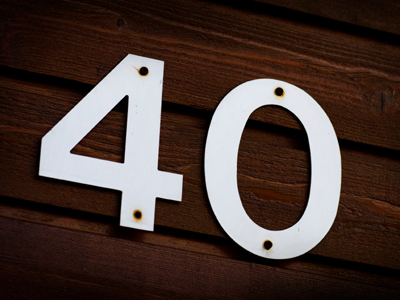10 x 4 = 40.

# 4 Times Table

This Math quiz is called '4 Times Table' and it has been written by teachers to help you if you are studying the subject at elementary school. Playing educational quizzes is an enjoyable way to learn if you are in the 3rd, 4th or 5th grade - aged 8 to 11.

It costs only \$12.50 per month to play this quiz and over 3,500 others that help you with your school work. You can subscribe on the page at Join Us

Learning the 4 times table is all about multiplying numbers by 4. One way of learning your times tables is to practice them over and over again until you know them by heart, this will help you in later life when you will have to multiply numbers more often than you think!

Test how well you know your 4 times table by playing the following quiz.

1.
8 x 4 =
28
30
32
38
8 x 4 can be written as either 8 + 8 + 8 + 8 or 4 + 4 + 4 + 4 + 4 + 4 + 4 + 4
2.
7 x 4 =
21
25
28
30
3.
12 x 4 =
16
40
46
48
4.
9 x 4 =
30
32
36
40
5.
11 x 4 =
4
40
42
44
To multiply a number by 11 just times it by 10 and then add it again, so 4 x 10 = 40, 40 + 4 = 44
6.
2 x 4 =
4
6
8
12
To multiply a number by 2 just double it
7.
4 x 4 =
8
16
32
36
8.
5 x 4 =
10
16
20
30
9.
6 x 4 =
12
24
26
28
To times a number by 6, first times it by 10, next half it and finally add the first number once more. So, 4 x 10 = 40, 40 ÷ 2 = 20 and 20 + 4 = 24
10.
3 x 4 =
6
12
15
18
There are 12 people on every jury
Author:  Amanda Swift